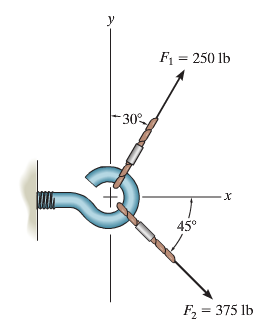# Problem: Determine the magnitude of the resultant force FR = F1 + F2 and its direction, measured counterclockwise from the positive x-axis.

###### FREE Expert Solution

Vector Magnitude:

$\overline{)\mathbf{|}\stackrel{\mathbf{⇀}}{\mathbit{A}}\mathbf{|}{\mathbf{=}}\sqrt{{{\mathbit{A}}_{\mathbit{x}}}^{\mathbf{2}}\mathbf{+}{{\mathbit{A}}_{\mathbit{y}}}^{\mathbf{2}}}}$

Direction:

$\overline{){\mathbf{tan}}{\mathbit{\theta }}{\mathbf{=}}\frac{{\mathbit{A}}_{\mathbit{y}}}{{\mathbit{A}}_{\mathbit{x}}}}$

In the y-direction:

Fy = F1cos(30°) - F2 sin(45°)

Fy = (250)cos(30°) - (375)sin(45°) = - 48.66 lb

82% (369 ratings)###### Problem Details

Determine the magnitude of the resultant force FR = F1 + F2 and its direction, measured counterclockwise from the positive x-axis.